Contents >> Applied Mathematics >> Matrix Algebra >> Principles of Matrix Calculation >> The transposed matrix

 Matrix Algebra - The transposed matrix The transposed matrix Replacement of rows by columns in a matrix of dimension m × n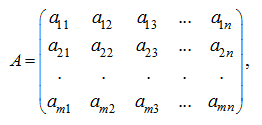gives the so-called transposed matrix of dimension n × m :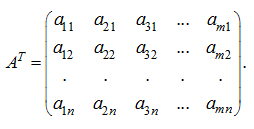In particular, for a vector-row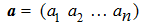the transposed matrix is the vector-columnThe basic properties of the transposed matrix: 1) twice transposed matrix coincides with initial matrix: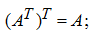2) the transposed matrix of the sum of matrices is equal to the sum of the transposed matrix addends, that is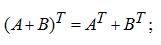3) the transposed matrix of the product of matrices is equal to the product of the transposed matrix factors, taken upside-down: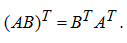For a square matrix the obvious equality takes place: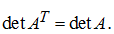If the matrix coincides with the transposed one, that is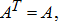then it is called symmetric . From this equality follows, that the symmetric matrix is square, and its elements are symmetric concerning the main diagonal, are equal among themselves: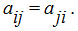Apparently, that the product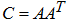is a symmetric matrix as, using property 3, we’ll receive: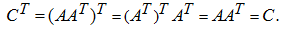E x a m p l e .  The matrix A and the transposed matrix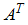are given: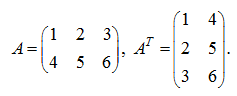Calculate the products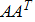and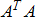. S o l u t i o n .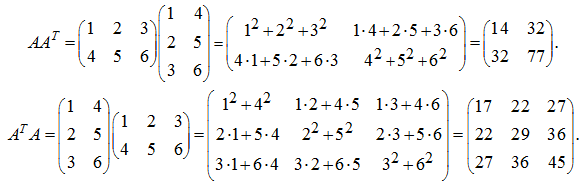As one would expect, the symmetric matrices have been received.

 < Previous Contents Next >